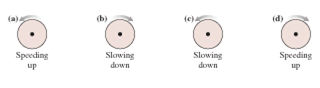# Problem: Figure 1 shows four rotating wheels. For each, determine the signs (+ or -)of ω and α.

###### FREE Expert Solution

α is the angular acceleration, defined as the rate of change of angular velocity, ω.

If the increase in ω is clockwise then the vector for α points away, that is, α is negative.

On the other hand, if ω is counterclockwise, then α points towards the observer, hence, positive.

80% (491 ratings)###### Problem Details

Figure 1 shows four rotating wheels. For each, determine the signs (+ or -)of ω and α.